# Math in Focus Grade 5 Chapter 2 Practice 7 Answer Key Order of Operations

Go through the Math in Focus Grade 5 Workbook Answer Key Chapter 2 Practice 7 Order of Operations to finish your assignments.

## Math in Focus Grade 5 Chapter 2 Practice 7 Answer Key Order of Operations

Simplify. Record each step.

Example
18 — 11 — 4 = 3
Step 1: 18 – 11 = 7
Step 2: 7 – 4 = 3
3,

Explanation:
The order of operation in math is a set of rules revolving around 4 major operators.
According to the order of operations, there is a particular sequence which we need to follow,
on each operator while solving the given mathematical expression we apply DMAS rule.
DMAS is the elementary rule for the order of operation of the Binary operations.
This States that Division will be done before Multiplication,
Step 1: 18 – 11 = 7,
Step 2 : 7 – 4 =3.

Question 1.
26 + 8 – 19 = __15__
Step 1 ______
Step 2 ______
15,

Explanation:
The order of operation in math is a set of rules revolving around 4 major operators.
According to the order of operations, there is a particular sequence which we need to follow,
on each operator while solving the given mathematical expression we apply DMAS rule.
DMAS is the elementary rule for the order of operation of the Binary operations.
This States that Division will be done before Multiplication,
Step 1 : 26 + 8 = 34,
Step 2 : 34 – 19 = 15.

Question 2.
12 + 16 – 9 + 3 = _22__
Step 1 ___
Step 2 ___
Step 3 ____
22,

Explanation:
The order of operation in math is a set of rules revolving around 4 major operators.
According to the order of operations, there is a particular sequence which we need to follow,
on each operator while solving the given mathematical expression we apply DMAS rule.
DMAS is the elementary rule for the order of operation of the Binary operations.
This States that Division will be done before Multiplication,
Step 1:   12 + 16 = 28,
Step 2 : 28 + 3 = 31,
Step 3 :  31 – 9 = 22.

Question 3.
58 – 23 + 11 – 6 = _40__
Step 1 ___
Step 2 ___
Step 3 ____
40,

Explanation:
The order of operation in math is a set of rules revolving around 4 major operators.
According to the order of operations, there is a particular sequence which we need to follow,
on each operator while solving the given mathematical expression we apply DMAS rule.
DMAS is the elementary rule for the order of operation of the Binary operations.
This States that Division will be done before Multiplication,
Step 1: 58 + 11 = 69,
Step 2: 69 – 23 = 46,
Step 3: 46 – 6 = 40.

Simplify. State the order in which you performed the operations.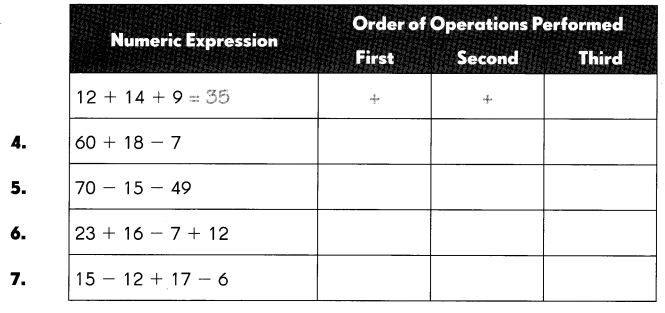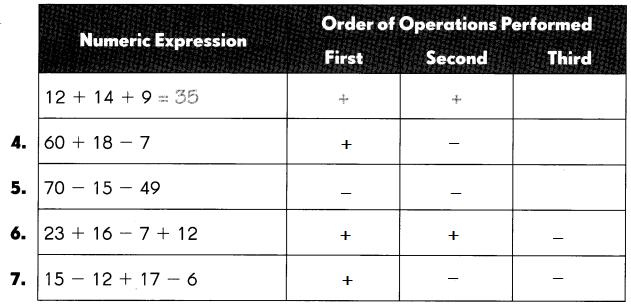Question 4.
60 + 18 – 7
71,

Explanation:
The order of operation in math is a set of rules revolving around 4 major operators.
According to the order of operations, there is a particular sequence which we need to follow,
on each operator while solving the given mathematical expression we apply DMAS rule.
DMAS is the elementary rule for the order of operation of the Binary operations.
This States that Division will be done before Multiplication,
Step 1: 60 + 18 = 78,
Step 2: 78 – 7 = 71.

Question 5.
70 – 15 – 49 = 6
6,

Explanation:
The order of operation in math is a set of rules revolving around 4 major operators.
According to the order of operations, there is a particular sequence which we need to follow,
on each operator while solving the given mathematical expression we apply DMAS rule.
DMAS is the elementary rule for the order of operation of the Binary operations.
This States that Division will be done before Multiplication,
Step 1: 70 – 49 = 21,
Step 2: 21 – 15 = 6.

Question 6.
23 + 16 – 7 + 12
44,

Explanation:
The order of operation in math is a set of rules revolving around 4 major operators.
According to the order of operations, there is a particular sequence which we need to follow,
on each operator while solving the given mathematical expression we apply DMAS rule.
DMAS is the elementary rule for the order of operation of the Binary operations.
This States that Division will be done before Multiplication,
Step 1: 23 + 16 = 39,
Step 2: 39 + 12 = 51,
Step 3: 51 – 7 = 44.

Question 7.
15 – 12 + 17 – 6
14,

Explanation:
The order of operation in math is a set of rules revolving around 4 major operators.
According to the order of operations, there is a particular sequence which we need to follow,
on each operator while solving the given mathematical expression we apply DMAS rule.
DMAS is the elementary rule for the order of operation of the Binary operations.
This States that Division will be done before Multiplication,
Step 1: 15 + 17 = 32,
Step 2: 32 – 12 = 20,
Step 3: 20 – 6 = 14.

Simplify. Record each step.

Example
9 × 6 ÷ 2 = 27
Step 1: 9 × 6 = 54
Step 2: 54 ÷ 2 = 27

Question 9.
200 ÷ 10 × 3 ÷ 5 = _12__
Step 1 _________
Step 2 _________
Step 3 _________
12,

Explanation:
According to the order of operations, there is a particular sequence which we need to follow,
on each operator while solving the given mathematical expression we apply DMAS rule.
DMAS is the elementary rule for the order of operation of the Binary operations.
This States that Division will be done before Multiplication,
Step 1: 200 ÷ 10 = 20,
Step 2: 20 ÷ 5 = 4,
Step 3: 4 x 3 = 12.

Question 10.
250 ÷ 5 ÷ 10 × 2 = _10__
Step 1 _________
Step 2 _________
Step 3 _________
10,

Explanation:
According to the order of operations, there is a particular sequence which we need to follow,
on each operator while solving the given mathematical expression we apply DMAS rule.
DMAS is the elementary rule for the order of operation of the Binary operations.
This States that Division will be done before Multiplication,
Step 1: 250 ÷ 10 = 25,
Step 2: 25 ÷ 5 = 5,
Step 3: 5 x 2 = 10.

Simplify. State the order in which you performed the operations.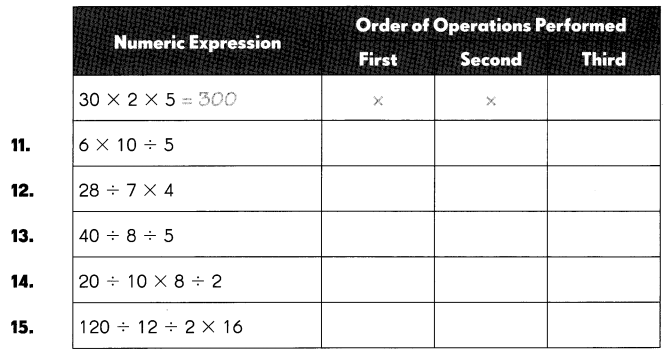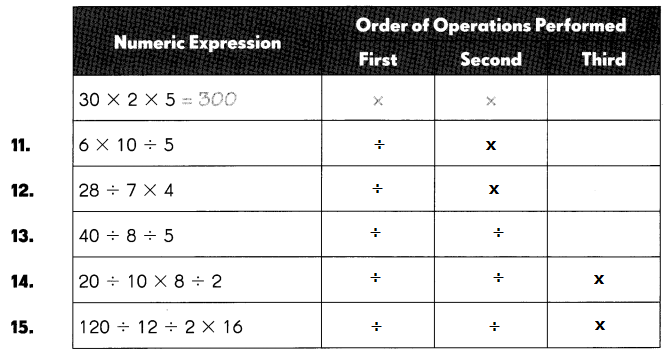Question 11.
6 × 10 ÷ 5
12,

Explanation:
According to the order of operations, there is a particular sequence which we need to follow,
on each operator while solving the given mathematical expression we apply DMAS rule.
DMAS is the elementary rule for the order of operation of the Binary operations.
This States that Division will be done before Multiplication,
Step 1: 10 ÷  5 = 2,
Step 2: 6 x 2 = 12.

Question 12.
28 ÷ 7 × 4
16,

Explanation:
According to the order of operations, there is a particular sequence which we need to follow,
on each operator while solving the given mathematical expression we apply DMAS rule.
DMAS is the elementary rule for the order of operation of the Binary operations.
This States that Division will be done before Multiplication,
Step 1: 28 ÷  7 = 4,
Step 2: 4 x 4 = 16.

Question 13.
40 ÷ 8 ÷ 5
1,

Explanation:
According to the order of operations, there is a particular sequence which we need to follow,
on each operator while solving the given mathematical expression we apply DMAS rule.
DMAS is the elementary rule for the order of operation of the Binary operations.
This States that Division will be done before Multiplication,
Step 1: 40 ÷  8 = 5,
Step 2: 5 ÷ 5 = 1.

Question 14.
20 ÷ 10 × 8 ÷ 2
8,

Explanation:
According to the order of operations, there is a particular sequence which we need to follow,
on each operator while solving the given mathematical expression we apply DMAS rule.
DMAS is the elementary rule for the order of operation of the Binary operations.
This States that Division will be done before Multiplication,
Step 1: 20 ÷  10 = 2,
Step 2: 2 ÷  2 = 1,
Step 3: 1 x 8 = 8.

Question 15.
120 ÷ 12 ÷ 2 × 16
80,

Explanation:
According to the order of operations, there is a particular sequence which we need to follow,
on each operator while solving the given mathematical expression we apply DMAS rule.
DMAS is the elementary rule for the order of operation of the Binary operations.
This States that Division will be done before Multiplication,

Step 1:
120 ÷  12 = 10,
Step 2:
10 ÷ 2 = 5,
Step 3:
16 x 5 = 80.

Simplify. Record each step.

Example
7 × 8 – 6=50
Step 1: 7 × 8 = 56
Step 2: 56 – 6 = 50

Question 16.
14 + 9 × 7 = __77__
Step 1 ___
Step 2 ____
77,

Explanation:
According to the order of operations, there is a particular sequence which we need to follow,
on each operator while solving the given mathematical expression we apply DMAS rule.
DMAS is the elementary rule for the order of operation of the Binary operations.
This States that Division will be done before Multiplication,
Step 1:  9 x 7 = 63,
Step 2:  63 + 14 = 77.

Question 17.
200 ÷ 20 + 5 = _15__
Step 1 ___
Step 2 ____
15,

Explanation:
According to the order of operations, there is a particular sequence which we need to follow,
on each operator while solving the given mathematical expression we apply DMAS rule.
DMAS is the elementary rule for the order of operation of the Binary operations.
This States that Division will be done before Multiplication,
Step 1 : 200 ÷ 20 = 10,
Step 2 : 10 + 5 = 15.

Question 18.
80 – 16 ÷ 4 = _76__
Step 1 ___
Step 2 ____
76,

Explanation:
According to the order of operations, there is a particular sequence which we need to follow,
on each operator while solving the given mathematical expression we apply DMAS rule.
DMAS is the elementary rule for the order of operation of the Binary operations.
This States that Division will be done before Multiplication,
Step 1:
16 ÷ 4 = 4,
Step 2:  80 – 4 = 76.

Simplify. State the order in which you performed the operations.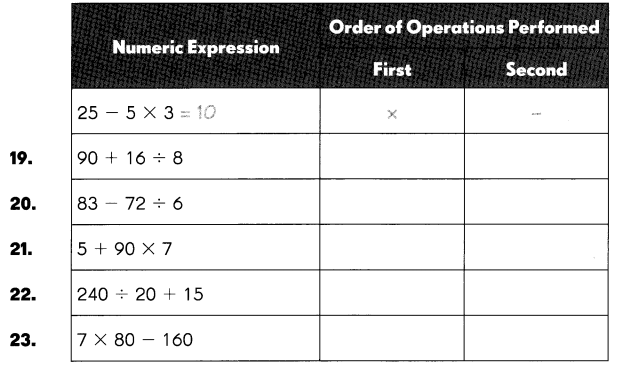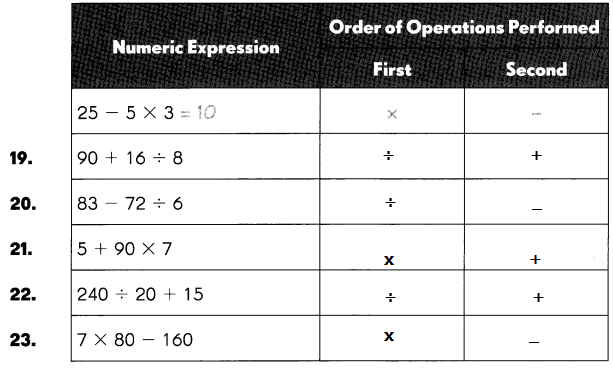Question 19.
90 + 16 ÷ 8
92,

Explanation:
According to the order of operations, there is a particular sequence which we need to follow,
on each operator while solving the given mathematical expression we apply DMAS rule.
DMAS is the elementary rule for the order of operation of the Binary operations.
This States that Division will be done before Multiplication,
Step 1: 16 ÷ 8 = 2,
Step 2: 90 + 2 = 92.

Question 20.
83 – 72 ÷ 6
71,

Explanation:
According to the order of operations, there is a particular sequence which we need to follow,
on each operator while solving the given mathematical expression we apply DMAS rule.
DMAS is the elementary rule for the order of operation of the Binary operations.
This States that Division will be done before Multiplication,
Step 1: 72 ÷ 6 = 12,
Step 2: 83 – 12 = 71.

Question 21.
5 + 90 × 7
635,

Explanation:
According to the order of operations, there is a particular sequence which we need to follow,
on each operator while solving the given mathematical expression we apply DMAS rule.
DMAS is the elementary rule for the order of operation of the Binary operations.
This States that Division will be done before Multiplication,
Step 1: 90 x 7 = 630,
Step 2: 630 + 5 = 635.

Question 22.
240 ÷ 20 + 15
27,

Explanation:
According to the order of operations, there is a particular sequence which we need to follow,
on each operator while solving the given mathematical expression we apply DMAS rule.
DMAS is the elementary rule for the order of operation of the Binary operations.
This States that Division will be done before Multiplication,
Step 1: 240 ÷  20 = 12,
Step 2: 12 + 15 = 27.

Question 23.
7 × 80 – 160
400,

Explanation:
According to the order of operations, there is a particular sequence which we need to follow,
on each operator while solving the given mathematical expression we apply DMAS rule.
DMAS is the elementary rule for the order of operation of the Binary operations.
This States that Division will be done before Multiplication,
Step 1: 80 x 7 = 560,
Step 2: 560 – 160 = 400.

Simplify. Record each step.

Example
54 ÷ 6 + 20 × 4 = 89
Step 1: 54 ÷ 6 = 9
Step 2: 20 × 4 = 80
Step 3: 9 + 80 = 89

Question 24.
40 – 6 + 10 × 3 = _______
Step 1 __________________
Step 2 ________________
Step 3 _____________
64,

Explanation:
According to the order of operations, there is a particular sequence which we need to follow,
on each operator while solving the given mathematical expression we apply DMAS rule.
DMAS is the elementary rule for the order of operation of the Binary operations.
This States that Division will be done before Multiplication,
Step 1: 10 x 3 = 30,
Step 2: 30 + 40 = 70,
Step 3: 70 – 6 = 64.

Question 25.
36 ÷ 6 – 25 ÷ 5 = _______
Step 1 ________________
Step 2 ________________
Step 3 ___________________
1,

Explanation:
According to the order of operations, there is a particular sequence which we need to follow,
on each operator while solving the given mathematical expression we apply DMAS rule.
DMAS is the elementary rule for the order of operation of the Binary operations.
This States that Division will be done before Multiplication,
Step 1: 36 ÷  6 = 6,
Step 2: 25 ÷ 5 = 5,
Step 3: 6 – 5 = 1.

Question 26.
25 × 4 – 36 ÷ 9 = ___
Step 1 __________
Step 2 __________
Step 3 ________
96,

Explanation:
According to the order of operations, there is a particular sequence which we need to follow,
on each operator while solving the given mathematical expression we apply DMAS rule.
DMAS is the elementary rule for the order of operation of the Binary operations.
This States that Division will be done before Multiplication,
Step 1: 36 ÷  9 = 4,
Step 2: 25 x 4 = 100,
Step 3: 100 – 4 = 96.

Simplify. State the order in which you performed the operations.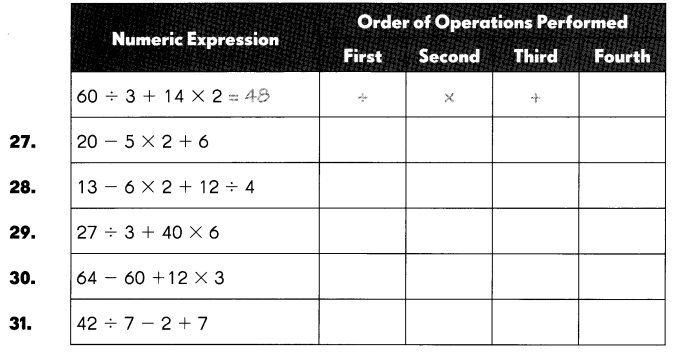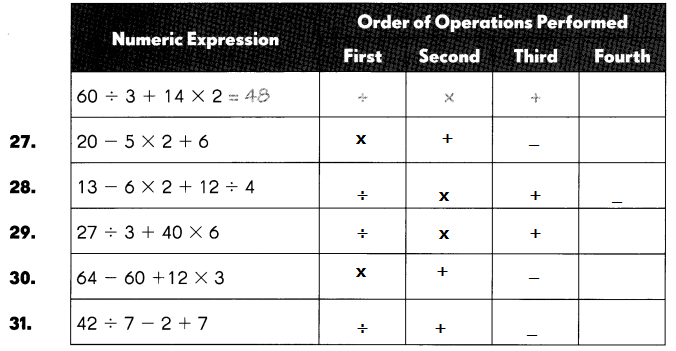Question 27.
20 – 5 × 2 + 6
16,

Explanation:
According to the order of operations, there is a particular sequence which we need to follow,
on each operator while solving the given mathematical expression we apply DMAS rule.
DMAS is the elementary rule for the order of operation of the Binary operations.
This States that Division will be done before Multiplication,
Step 1: 5 x 2 = 10,
Step 2: 20 – 10 = 10,
Step 3:  10 + 6 = 16.

Question 28.
13 – 6 × 2 + 12 ÷ 4
4,

Explanation:
According to the order of operations, there is a particular sequence which we need to follow,
on each operator while solving the given mathematical expression we apply DMAS rule.
DMAS is the elementary rule for the order of operation of the Binary operations.
This States that Division will be done before Multiplication,
Step 1: 12 ÷ 4 = 3,
Step 2: 6 x 2 = 12,
Step 3: 13 – 12 = 1,
Step 4: 3 + 1 = 4.

Question 29.
27 ÷ 3 + 40 × 6
249,

Explanation:
According to the order of operations, there is a particular sequence which we need to follow,
on each operator while solving the given mathematical expression we apply DMAS rule.
DMAS is the elementary rule for the order of operation of the Binary operations.
This States that Division will be done before Multiplication,
Step 1: 27 ÷ 3 = 9,
Step 2: 40 x 6 = 240,
Step 3: 240 + 9 = 249.

Question 30.
64 – 60 + 12 × 3
40,

Explanation:
According to the order of operations, there is a particular sequence which we need to follow,
on each operator while solving the given mathematical expression we apply DMAS rule.
DMAS is the elementary rule for the order of operation of the Binary operations.
This States that Division will be done before Multiplication,
Step 1: 12 x 3 = 36,
Step 2: 36 + 64 = 100,
Step 3: 100 – 60 =40.

Question 31.
42 ÷ 7 – 2 + 7
11,

Explanation:
According to the order of operations, there is a particular sequence which we need to follow,
on each operator while solving the given mathematical expression we apply DMAS rule.
DMAS is the elementary rule for the order of operation of the Binary operations.
This States that Division will be done before Multiplication,
Step 1: 42 ÷ 7 = 6,
Step 2: 6 + 7 = 13,
Step 3: 13  – 2 = 11.

Simplify. Record each step.

Example
(15 – 11) × 9
Step 1: 15 – 11 = 4
Step 2: 4 × 9 = 36

Question 32.
(11 + 5) ÷ 16 = _1__
Step 1 ____
Step 2 ____
1,

Explanation:
According to the order of operations, there is a particular sequence which we need to follow,
on each operator while solving the given mathematical expression we use BODMAS rule.
The BODMAS stands for
B – Brackets, O – Order of powers, D – Division, M – Multiplication, A – Addition, and S – Subtraction.
Step 1: 11 + 5 = 16,
Step 2: 16 ÷ 16 = 1.

Simplify. Record each step.

Question 33.
63 – (9 × 7) = _0_
Step 1 ______
Step 2 ________________
0,

Explanation:
According to the order of operations, there is a particular sequence which we need to follow,
on each operator while solving the given mathematical expression we apply BODMAS rule.
The acronym stands for
B – Brackets, O – Order of powers, D – Division, M – Multiplication, A – Addition and S – Subtraction.
Step 1: 9 x 7 = 63,
Step 2: 63 – 63 = 0.

Question 34.
32 ÷ (14 + 2) = ____
Step 1 __________________
Step 2 __________________
2,

Explanation:
According to the order of operations, there is a particular sequence which we need to follow,
on each operator while solving the given mathematical expression we apply BODMAS rule.
The acronym stands for
B – Brackets, O – Order of powers, D – Division, M – Multiplication, A – Addition and S – Subtraction.
Step 1 : 14 + 2 = 16,
Step 2 : 32 ÷  16 = 2.

Simplify. State the order in which you performed the operations.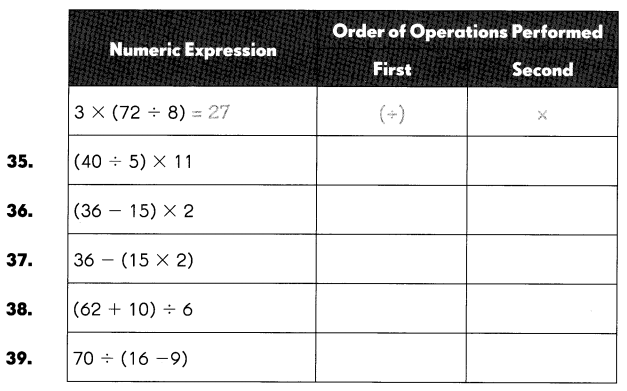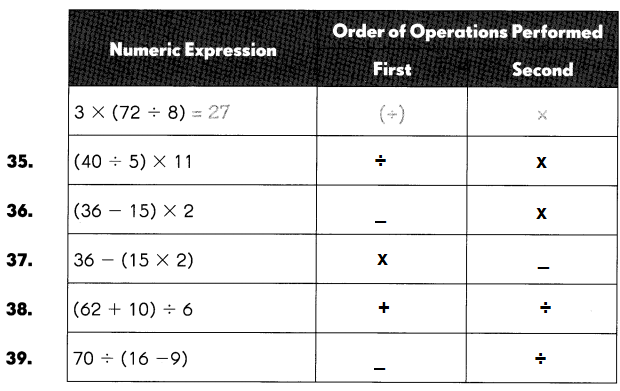Question 35.

(40 ÷ 5) × 11
88,

Explanation:
According to the order of operations, there is a particular sequence which we need to follow,
on each operator while solving the given mathematical expression we apply BODMAS rule.
BODMAS is the elementary rule for the order of operation of the Binary operations.
The acronym stands for B – Brackets, O – Order of powers, D – Division,
M – Multiplication, A – Addition, and S – Subtraction.
Step 1 40 ÷  5 = 8,
Step 2 8 x 11 = 88.

Question 36.
(36 – 15) × 2
42,

Explanation:
According to the order of operations, there is a particular sequence which we need to follow,
on each operator while solving the given mathematical expression we apply BODMAS rule.
BODMAS is the elementary rule for the order of operation of the Binary operations.
The acronym stands for B – Brackets, O – Order of powers, D – Division,
M – Multiplication, A – Addition, and S – Subtraction,
Step 1: 36 – 15 = 21,
Step 2: 21 x 2 = 42.

Question 37.
36 – (15 × 2)
6,

Explanation:
According to the order of operations, there is a particular sequence which we need to follow,
on each operator while solving the given mathematical expression we apply BODMAS rule.
BODMAS is the elementary rule for the order of operation of the Binary operations.
The acronym stands for
B – Brackets, O – Order of powers, D – Division, M – Multiplication,
A – Addition, and S – Subtraction.,
Step 1: 15 x 2 = 30,
Step 2: 36 – 30 = 6.

Question 38.
(62 + 10) ÷ 6
12,

Explanation:
According to the order of operations, there is a particular sequence which we need to follow,
on each operator while solving the given mathematical expression we apply BODMAS rule.
BODMAS is the elementary rule for the order of operation of the Binary operations.
The acronym stands for
B – Brackets, O – Order of powers, D – Division, M – Multiplication,
A – Addition, and S – Subtraction,
Step 1:
62 + 10 = 72,
Step 2:
72 ÷  6 =12.

Question 39.
70 ÷ (16 – 9)
10,

Explanation:
According to the order of operations, there is a particular sequence which we need to follow,
on each operator while solving the given mathematical expression we apply BODMAS rule.
BODMAS is the elementary rule for the order of operation of the Binary operations.
The acronym stands for B – Brackets, O – Order of powers, D – Division,
M – Multiplication, A – Addition, and S – Subtraction.

Step 1:
16 – 9 = 7,
Step 2 :
70 ÷  7 = 10.

Simplify. Record each step.

Example
21 + (12 + 6) ÷ 3 = 27
Step 1 12 + 6 = 18
Step 2 18 ÷ 3 = 6
Step 3 21 + 6 = 27

Question 40.
7 + (8 – 4) × 10 = _47___
Step 1 _______________
Step 2 _________
Step 3 ______
47,

Explanation:
According to the order of operations, there is a particular sequence which we need to follow,
on each operator while solving the given mathematical expression we apply BODMAS rule.
BODMAS is the elementary rule for the order of operation of the Binary operations.
The acronym stands for B – Brackets, O – Order of powers, D – Division,
M – Multiplication, A – Addition, and S – Subtraction.
Step 1 : 8 – 4 = 4,
Step 2 : 4 x 10 = 40,
Step 3 : 40 + 7 = 47.

Question 41.
32 ÷ (7 + 1) × 9 – 5 = ____
Step 1 _______________
Step 2 _________
Step 3 ______
Step 4 ______
31,

Explanation:
According to the order of operations, there is a particular sequence which we need to follow,
on each operator while solving the given mathematical expression we apply BODMAS rule.
BODMAS is the elementary rule for the order of operation of the Binary operations.
The acronym stands for B – Brackets, O – Order of powers, D – Division, M – Multiplication,
A – Addition, and S – Subtraction.
Step 1  :  7 + 1 = 8
Step 2 : 32  ÷  8 = 4
Step 3 : 4 x 9 = 36
Step 4 : 36 – 5 = 31.

Simplify. Record each step.

Question 42.
(47 + 12) – 10 ÷ 5 × 3 = ___
Step 1 _________________
Step 2 ______________
Step 3 __________________
Step 4 __________________
53,

Explanation:
According to the order of operations, there is a particular sequence which we need to follow,
on each operator while solving the given mathematical expression we apply BODMAS rule.
BODMAS is the elementary rule for the order of operation of the Binary operations.
The acronym stands for B – Brackets, O – Order of powers, D – Division,
M – Multiplication, A – Addition, and S – Subtraction.
Step 1: 47 + 12 = 59,
Step 2:  10 ÷ 5 = 2,
Step 3: 2 x 3 =6,
Step 4: 59 – 6 = 53.

Simplify. State the order in which you performed the operations.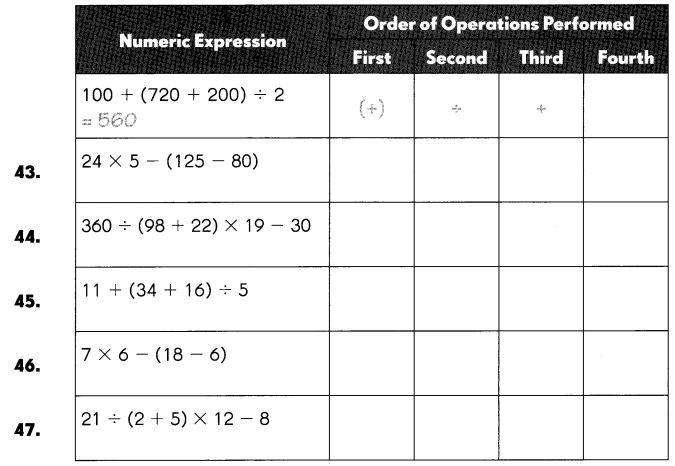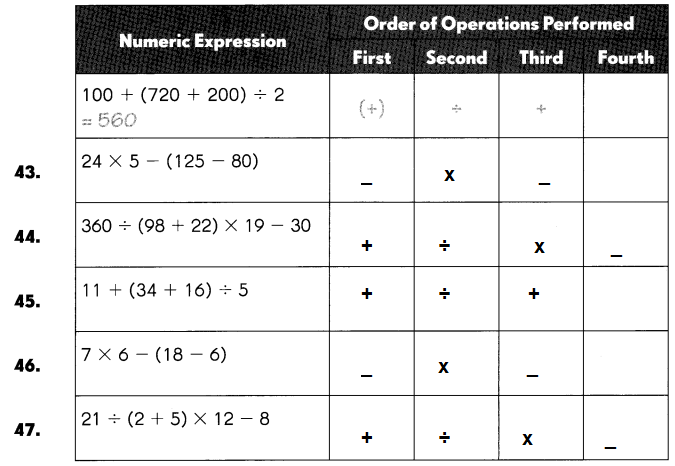Question 43.
24 × 5 – (125 – 80)
75,

Explanation:
According to the order of operations, there is a particular sequence which we need to follow,
on each operator while solving the given mathematical expression we apply BODMAS rule.
BODMAS is the elementary rule for the order of operation of the Binary operations.
The acronym stands for B – Brackets, O – Order of powers,
D – Division, M – Multiplication, A – Addition, and S – Subtraction.
Step 1 : 125 – 80 = 45,
Step 2 : 24 x 5 = 120,
Step 3: 120 – 45 = 75.

Question 44.
360 ÷ (98 + 22) × 19 – 30
27,

Explanation:
According to the order of operations, there is a particular sequence which we need to follow,
on each operator while solving the given mathematical expression we apply BODMAS rule.
BODMAS is the elementary rule for the order of operation of the Binary operations.
The acronym stands for B – Brackets, O – Order of powers,
D – Division, M – Multiplication, A – Addition, and S – Subtraction.
Step 1: 98 + 22 = 120,
Step 2: 360 ÷ 120 = 3,
Step 3: 3 x 19 = 57,
Step 4: 57 – 30 = 27.

Question 45.
11 + (34 + 16) ÷ 5
21,

Explanation:
According to the order of operations, there is a particular sequence which we need to follow,
on each operator while solving the given mathematical expression we apply BODMAS rule.
BODMAS is the elementary rule for the order of operation of the Binary operations.
The acronym stands for B – Brackets, O – Order of powers, D – Division,
M – Multiplication, A – Addition, and S – Subtraction.
Step 1: 34 + 16 = 50,
Step 2: 50 ÷ 5 = 10,
Step 3: 10 + 11 = 21.

Question 46.
7 × 6 – (18 – 6)
30,

Explanation:
According to the order of operations, there is a particular sequence which we need to follow,
on each operator while solving the given mathematical expression we apply BODMAS rule.
BODMAS is the elementary rule for the order of operation of the Binary operations.
The acronym stands for B – Brackets, O – Order of powers, D – Division,
M – Multiplication, A – Addition, and S – Subtraction.
Step 1: 18 – 6 = 12,
Step 2: 7 x 6 = 42,
Step 3: 42 – 12 = 30.

Question 47.
21 ÷ (2 + 5) × 12 – 8
28,

Explanation:
According to the order of operations, there is a particular sequence which we need to follow,
on each operator while solving the given mathematical expression we apply BODMAS rule.
BODMAS is the elementary rule for the order of operation of the Binary operations.
The acronym stands for B – Brackets, O – Order of powers, D – Division,
M – Multiplication, A – Addition, and S – Subtraction.
Step 1: 2 + 5 = 7,
Step 2: 21 ÷ 7 = 3,
Step 3: 3 x 12 = 36,
Step 4: 36 – 8 = 28.

Simplify. Record each step.

Example
{50 – [13 – (8 + 3)]} ÷ 4 = 12
Step 1 8 + 3
Step 2 13 – 11 = 2
Step 3 50 – 2 = 48</>
Step 4 48 ÷ 4 = 12

Question 48.
19 – [(18 + 2) – 6]
Step 1 __________________
Step 2 __________________
Step 3 __________________
Step 1 18 + 2 = 20
Step 2 20 – 6 = 14
Step 3 19 – 14 = 5
Explanation:
According to the order of operations, there is a particular sequence which we need to follow,
on each operator while solving the given mathematical expression we apply BODMAS rule.
BODMAS is the elementary rule for the order of operation of the Binary operations.
The acronym stands for B – Brackets, O – Order of powers, D – Division, M – Multiplication, A – Addition, and S – Subtraction.

Question 49.
[(27 ÷ 9) – 3] + 30 = ___
Step 1 __________________
Step 2 __________________
Step 3 __________________
Step 1 27 ÷ 9 = 3
Step 2 3 – 3 = 0
Step 3 0 + 30 = 30
Explanation:
According to the order of operations, there is a particular sequence which we need to follow,
on each operator while solving the given mathematical expression we apply BODMAS rule.
BODMAS is the elementary rule for the order of operation of the Binary operations.
The acronym stands for B – Brackets, O – Order of powers, D – Division, M – Multiplication, A – Addition, and S – Subtraction.

Question 50.
11 + {18 – [15 ÷ (20 – 15)]}= ____
Step 1 __________________
Step 2 __________________
Step 3 __________________
Step 4 __________________
Step 1 20 – 15 = 5
Step 2 15 ÷ 5 = 3
Step 3 18 – 3 = 15
Step 4 11 + 15 = 26
Explanation:
According to the order of operations, there is a particular sequence which we need to follow,
on each operator while solving the given mathematical expression we apply BODMAS rule.
BODMAS is the elementary rule for the order of operation of the Binary operations.
The acronym stands for B – Brackets, O – Order of powers, D – Division, M – Multiplication, A – Addition, and S – Subtraction.

Question 51.
{[(100 ÷ 4) × (3 + 3)] ÷ 50} + 9 =
Step 1 ________________
Step 2 _____________
Step 3 __________________
Step 4 __________________
Step 5 __________________
Step 1 100 ÷ 4 = 25
Step 2 3 + 3 = 6
Step 3 2 5 x 6 = 150
Step 4 150 ÷ 50 = 3
Step 3 + 9 = 12
Explanation:
According to the order of operations, there is a particular sequence which we need to follow,
on each operator while solving the given mathematical expression we apply BODMAS rule.
BODMAS is the elementary rule for the order of operation of the Binary operations.
The acronym stands for B – Brackets, O – Order of powers, D – Division, M – Multiplication, A – Addition, and S – Subtraction.

Question 52.
(108 – 86) + {120 ÷ [20 – (10 + 6)]} = ___
Step 1 __________________
Step 2 ________________
Step 3 __________________
Step 4 __________________
Step 5 __________________